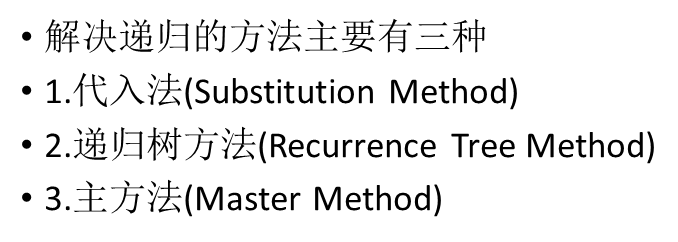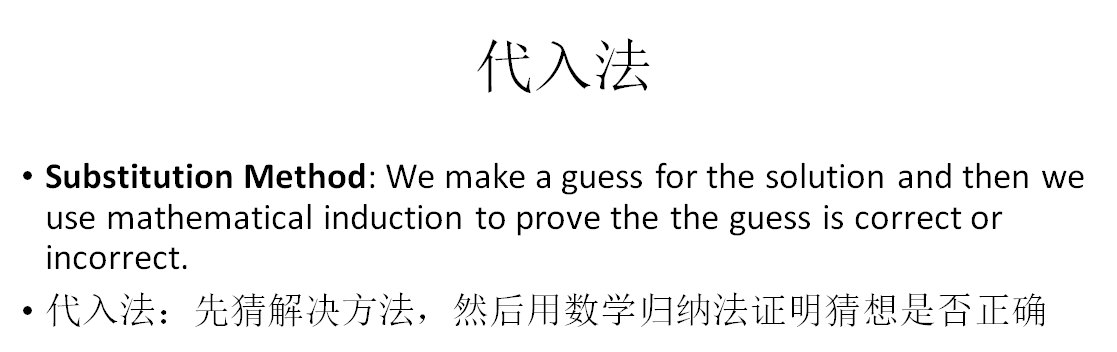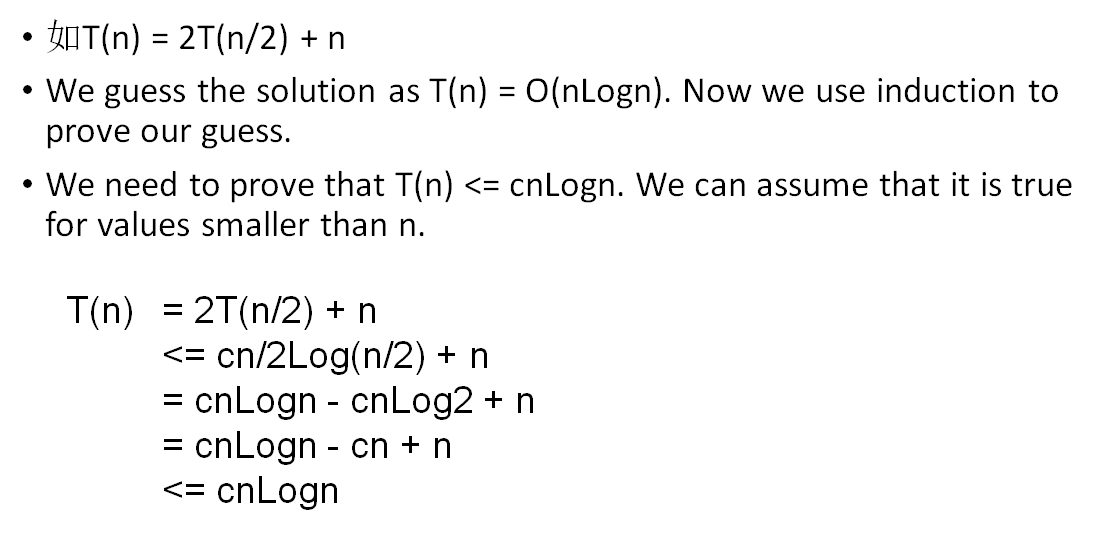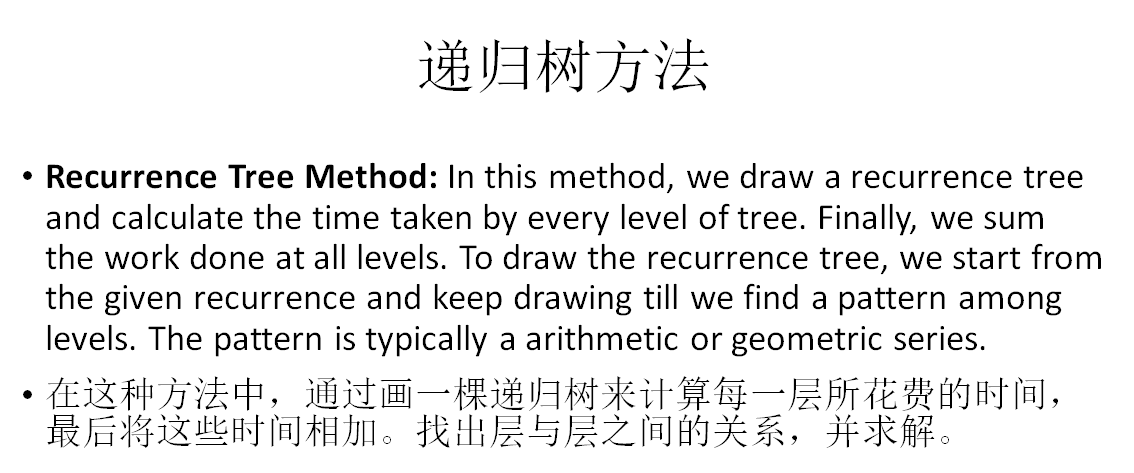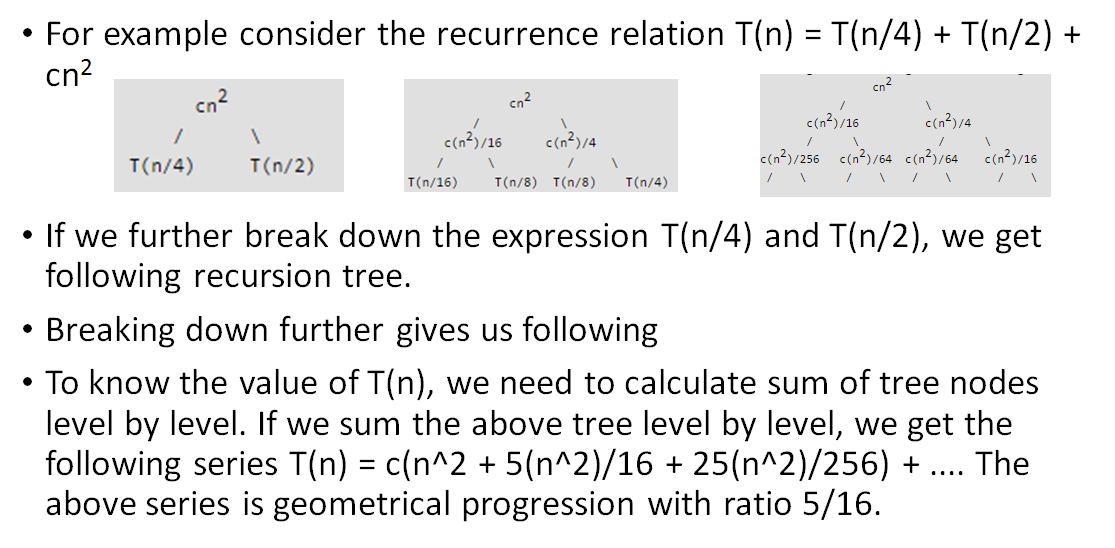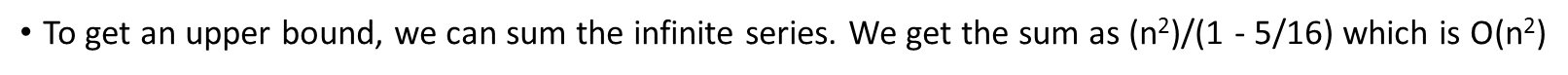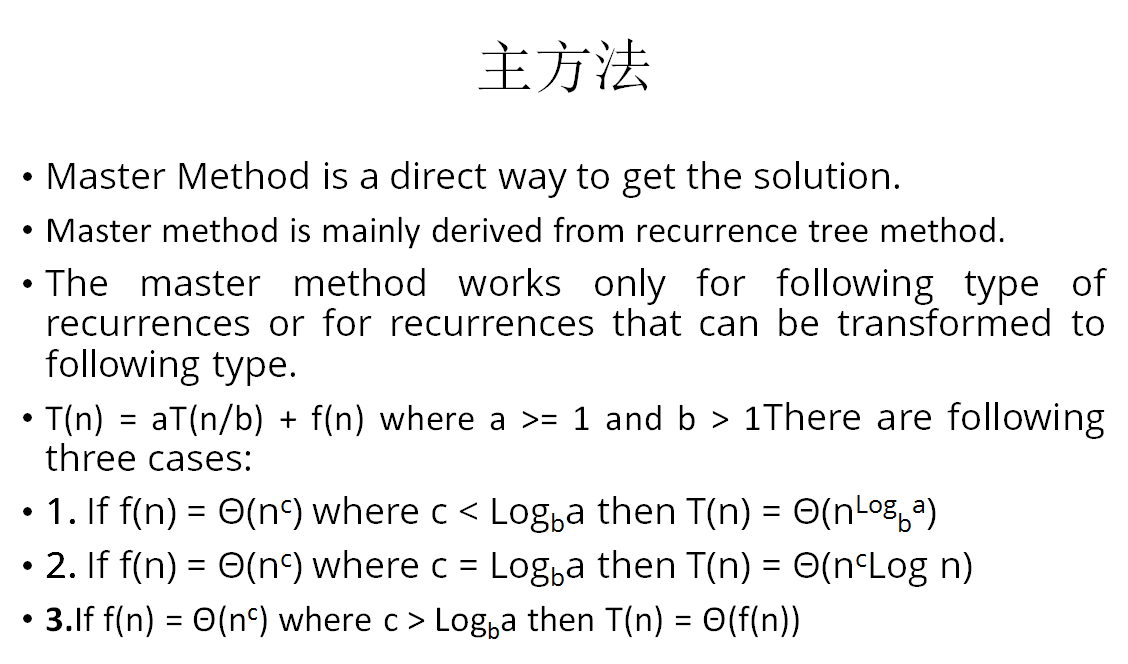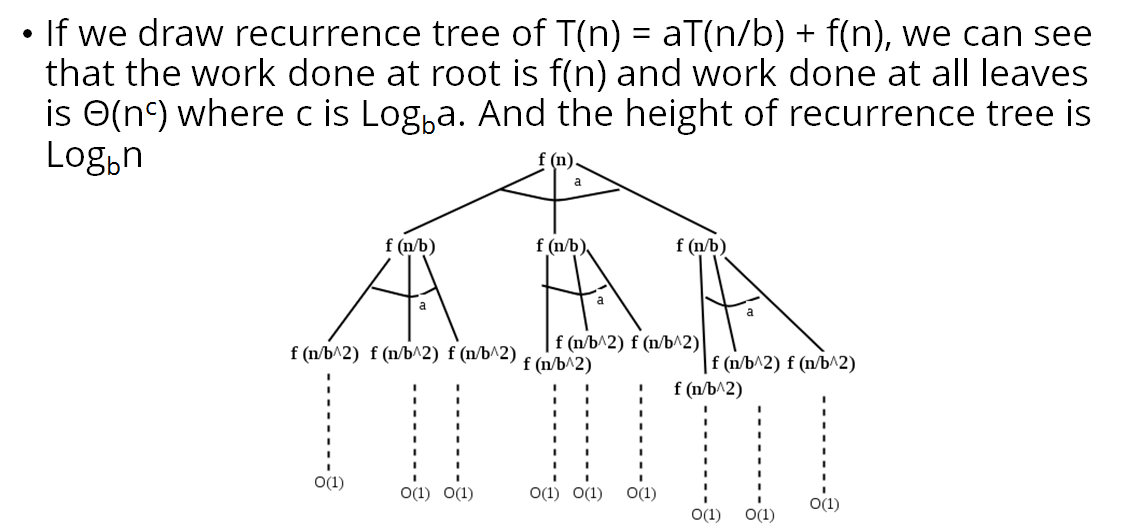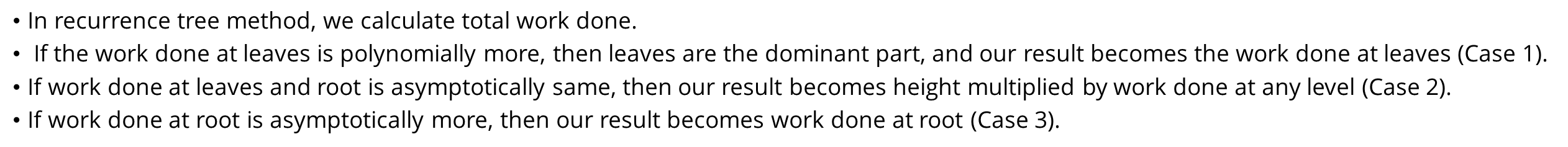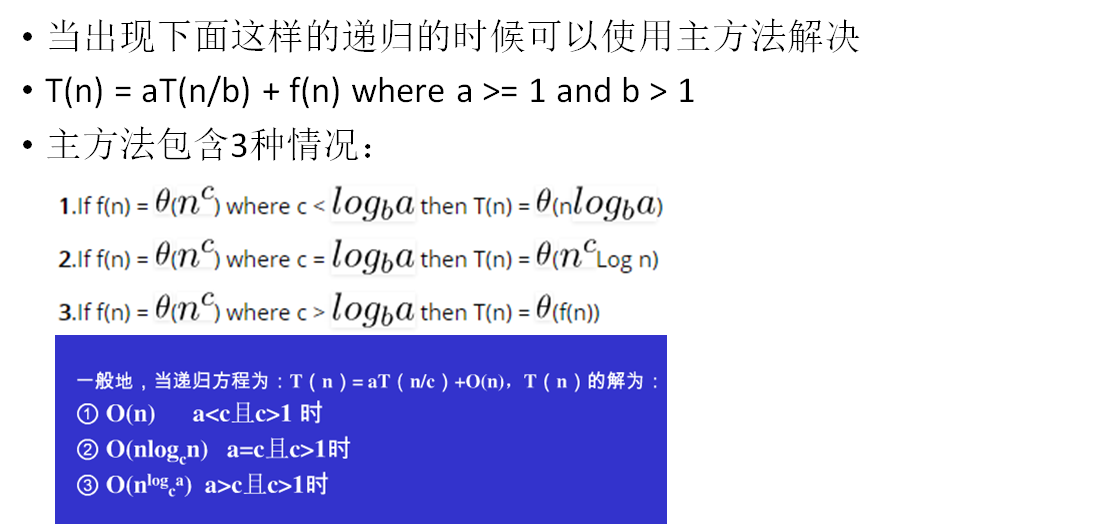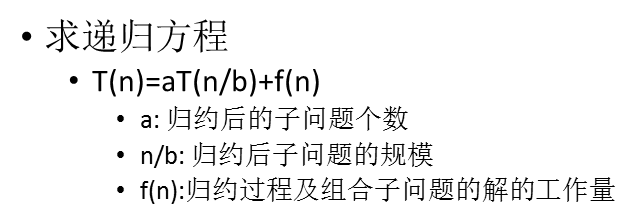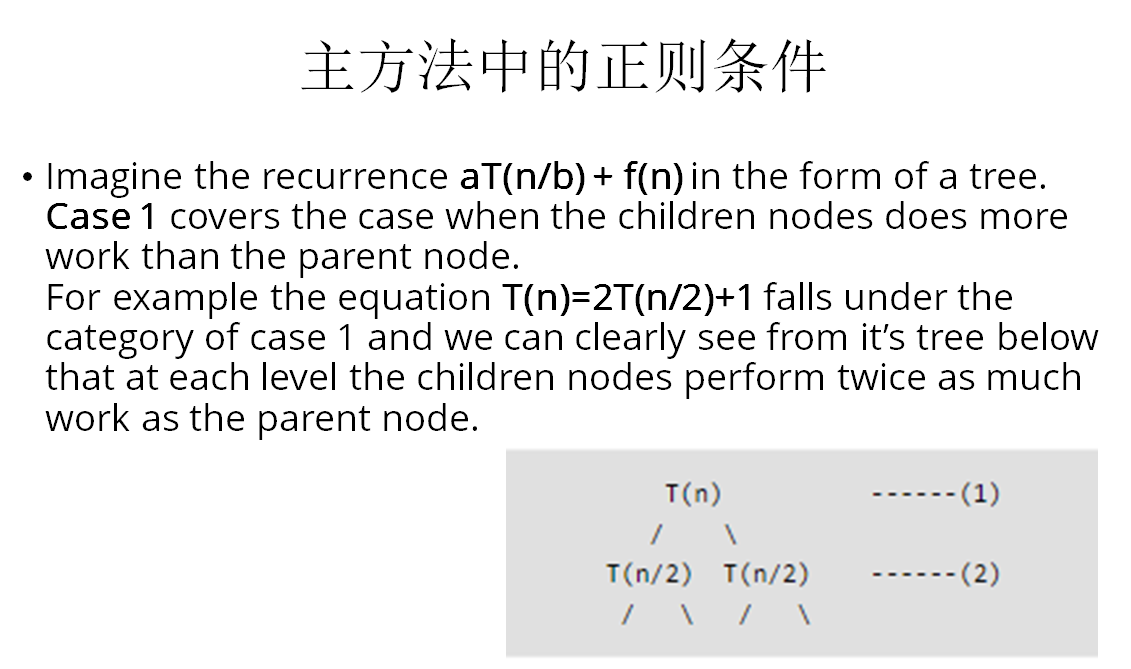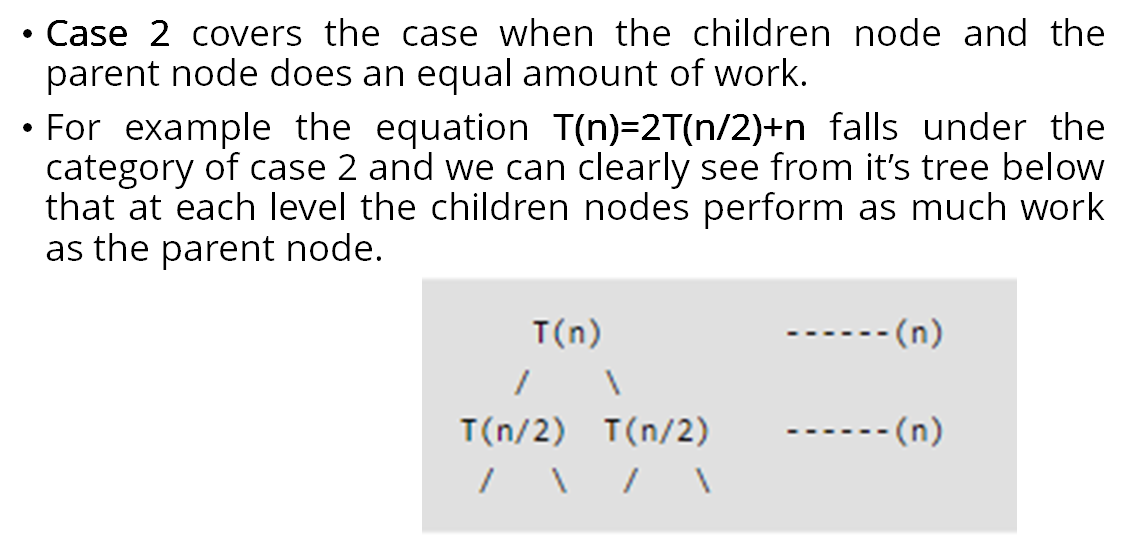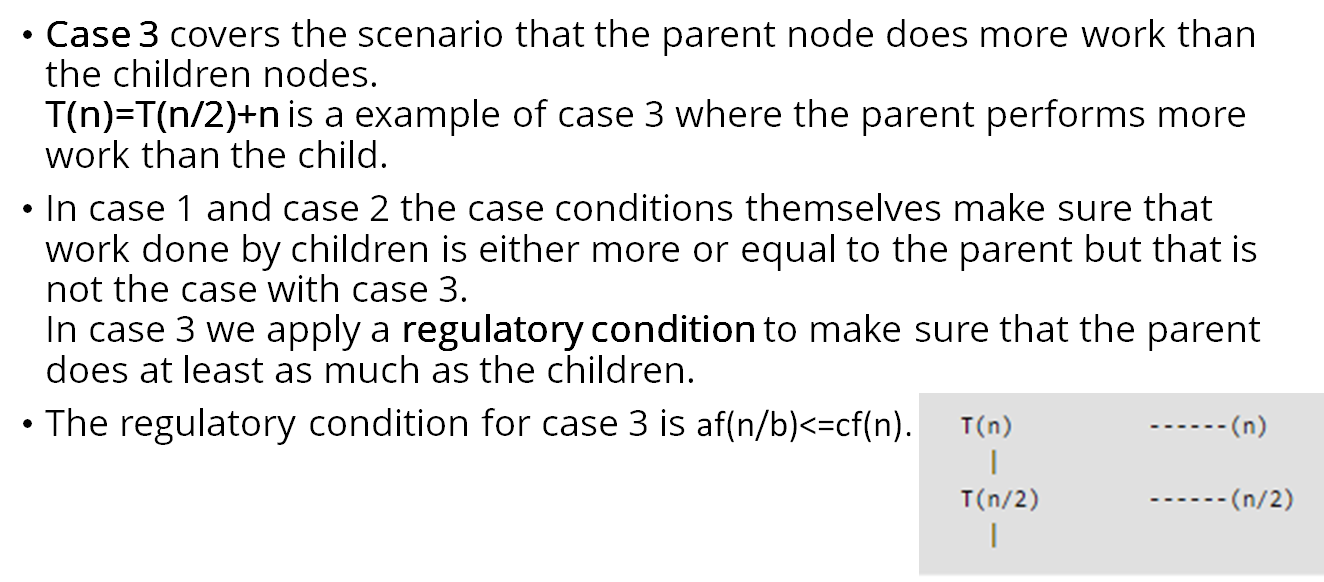# MwingFly

posts - 83, comments - 3, trackbacks - 0, articles - 0
::  ::  ::  ::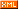::
• recursive algorithm is an algorithm which calls itself with "smaller (or simpler)" input values, and which obtains the result for the current input by applying simple operations to the returned value for the smaller (or simpler) input. More generally if a problem can be solved utilizing solutions to smaller versions of the same problem, and the smaller versions reduce to easily solvable cases, then one can use a recursive algorithm to solve that problem. For example, the elements of a recursively defined set, or the value of a recursively defined function can be obtained by a recursive algorithm.
• If a set or a function is defined recursively, then a recursive algorithm to compute its members or values mirrors the definition. Initial steps of the recursive algorithm correspond to the basis clause of the recursive definition and they identify the basis elements. They are then followed by steps corresponding to the inductive clause, which reduce the computation for an element of one generation to that of elements of the immediately preceding generation.
• In general, recursive computer programs require more memory and computation compared with iterative algorithms, but they are simpler and for many cases a natural way of thinking about the problem.